Select Page

Computer Organization

Memory Organization
Interrupt
DMA Controller

# Boolean Expressions & Functions

Boolean algebra developed by George Boole in 1847. Before to start the topic look at see the basic terms (i.e. Boolean Algebra, Boolean Expressions and Boolean Functions).

• A Boolean Algebra deals with binary variables (i.e. A, B, C), Binary Constants (0,1) and logic operation (i.e. NOT, AND, OR).
• Boolean Function is described by an algebraic expression called Boolean expression.
• Boolean Expression consists of binary variables, the constants (0 and 1), and the logic operation symbols.

###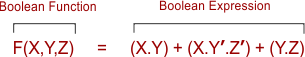The Boolean Expression is an INPUT and Boolean Function is an OUTPUT.

Note: Output of Boolean algebra function, on the given value of variables, can be either TRUE (1) or FALSE(0)  Let explain the following Boolean functions.

## Symbolic Representation Of Boolean Expressions/Functions

Symbolically the Boolean functions can be represented through Logic gates. Consider the function  F = (x.y) + (y’.z). The symbolically representation of this function is given under.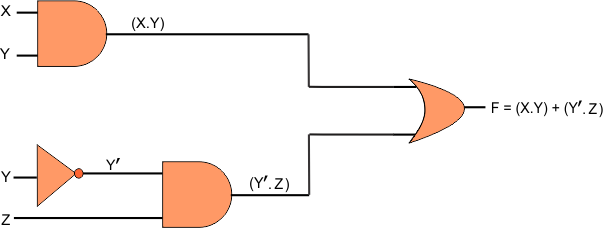## OUTPUT of Boolean Expressions/Functions

To find the output of any Boolean function, the Binary value of each variable is already given as a INPUT. SO, Simply replace the variables with their respective input values.

Let suppose a Boolean function  F(x,y,z) = (x.y) + (y’.z) and its input values are X=0, Y=1, Z=0 then the values of the Boolean function. The Output of given function is

``` F(x,y,z) = (x.y) + (y'.z)
As Y= 1 then, Y’ = 0
F(x,y,z)= (0.1) + (0.0)
F(x,y,z)= (0) + (0)
F(x,y,z)= 0```

## Truth Table of Boolean Function

To represent a function in a truth table, we require a list of the 2(N) combinations, where N is the number of binary variables. If there are 3 variables (x, y and z) then 2(3) = 8 combinations will required. For batter understanding about Boolean Truth table look read the topic of logic gates.

The truth table for the Boolean function F = (x.y) + (y’.z) is given under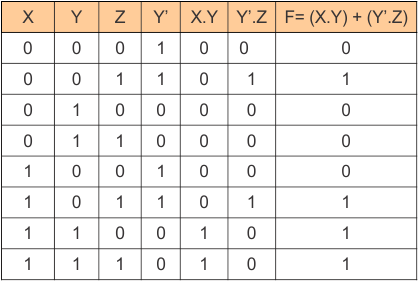## Boolean Functions with Truth Tables

Let explain some examples of Boolean Algebra Functions with their Truth Tables

### Example 01: F(X,Y)= (X+Y). Z

Block diagram for the Boolean function F(X,Y)= (X+Y). Z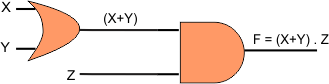The truth table for the Boolean function F(X,Y)= (X+Y). Z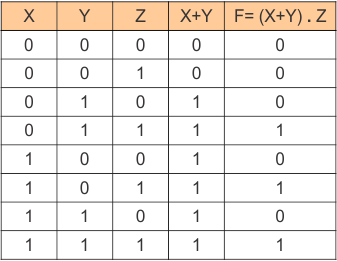### Example 02: F(X,Y)= (X’.Y’) + (X’.Z)

Block diagram for the Boolean function F(X,Y)= (X’.Y’) + (X’.Z)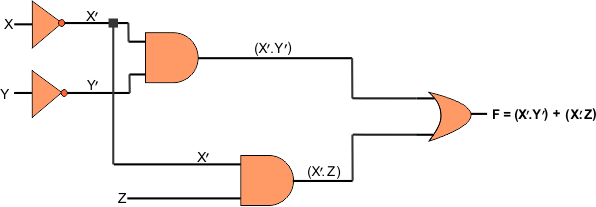The truth table for the Boolean function F(X,Y)= (X’.Y’) + (X’.Z)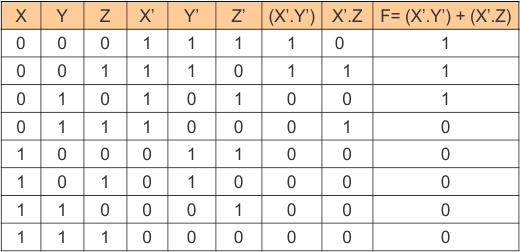### Example 03: F(X,Y) = (x’y’z) + (x’yz) + (xy’)

Block diagram for the Boolean function F(X,Y) = (x’y’z) + (x’yz) + (xy’)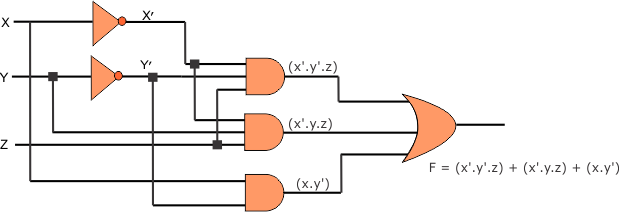The truth table for the Boolean function F(X,Y) = (x’y’z) + (x’yz) + (xy’)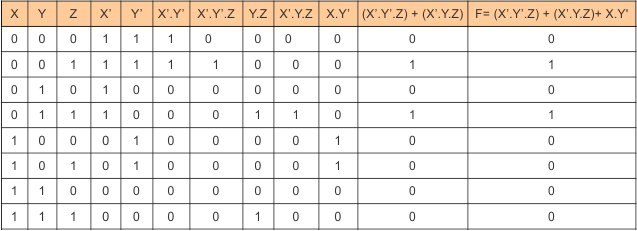Help Other’s By Sharing…